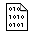## Firefly and PC GAMESS-related discussion club

Learn how to ask questions correctlyRe^3: Lack of force constants for bending vibrations in the output file

Wenli Zou
qcband@gmail.com

Hi Vyacheslav,

The force constants for stretching and bending internal coordinates can be calculated using the attached Fortran program, which is based on the methods described in J. Chem. Phys. 137, 084114 (2012). The original definitions of the adiabatic force constants and the adiabatic vibrational frequencies were described in Int. J. Quant. Chem. 67, 1 (1998); ibid. 67, 11 (1998).

It is valid for stationary points, and the geometry should be fully optimized using tight optimization convergence criteria. Otherwise, the force constant matrix in internal coordinates is not uniquely defined (for example, see J. Mol. Spectrosc. 205, 227 2001), and the calculated force constants will be isotope-dependent.

For bending or dihedral angles, two force constants are given, where Ka(d) is scaled by distants and Ka is not. Both of them were reported in literature.

Bending angle A-B-C:
Ka(d) = Ka / [R(A,B) * R(B,C)]

Dihedral angle A-B-C-D:
Ka(d) = Ka / [d(A,B-C) * d(D,B-C)]
= Ka * R(B,C) * R(B,C) / [4 * S(A,B,C) * S(B,C,D)]

d(A,B-C): distance between the atom A and the bond B-C
S(A,B,C): area of ​​the triangle ABC

To run the program, a modified PUNCH file from the Firefly frequency calculation is required. Please see the attached PUNCH file about the details.

Best regards,
Wenli

On Wed Sep 26 '12 12:47pm, Vyacheslav wrote
-------------------------------------------
>On Wed Sep 26 '12 1:08am, Alex Granovsky wrote
>----------------------------------------------
>>Hi,

>>how did you specify the z-matrix and a the value of nzvar variable?

>>Regards,
>>Alex Granovsky
>**************************************
>Hi,
>thanks for response.
>The first step is to optimize the structure. Then, in the input I enter the coordinates of the first calculation, internal coordinates in IZMAT and put in NZVAR the number equal to the number of internal coordinates given (for my structures it is always more than 3N-6). Format of coordinates in \$Data is irrelevant. One can enter a z-matrix or Cartesian coordinates (COORD=unique), the results are identical. In output file I get a complete set of intrinsic frequencies, while the force constants are for the stretching vibrations only.
>Example of such calculation is attached.
>Best regards,
>                 Vyacheslav

>This message contains the 38 kb attachment [ adia4f.tar.gz ] adiabatic force constants of internal coordinatesTue Oct 2 '12 0:35amThis message read 1178 times